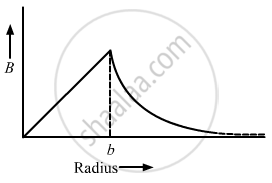Advertisement Remove all ads

# A Solid Wire of Radius 10 Cm Carries a Current of 5.0 a Distributed Uniformly Over Its Cross Section. Find the Magnetic Field B At a Point at a Distance - Physics

Short Note

A solid wire of radius 10 cm carries a current of 5.0 A distributed uniformly over its cross section. Find the magnetic field B at a point at a distance (a) 2 cm (b) 10 cm and (c) 20 cm away from the axis. Sketch a graph B versus x for 0 < x < 20 cm.

Advertisement Remove all ads

#### SolutionGiven:
Magnitude of current, i = 5 A
Radius of the wire, b$= 10 \text{ cm }= 10 \times {10}^{- 2}$  m

For a point at a distance a from the axis,
Current enclosed, $i' = \frac{i}{\pi b^2} \times \pi a^2$
By Ampere's circuital law,
$\oint B . dl = \mu_0 i'$
For the given conditions,

$B \times 2\pi a = \mu_0 \frac{i}{\pi b^2} \times \pi a^2$

$\Rightarrow B = \frac{\mu_0 ia}{2\pi b^2} \ldots\left( 1 \right)$

$(a)\text{ a = 2 cm }= 2 \times {10}^{- 2}$ m
Again, using the circuital law, we get

$B = \frac{4\pi \times {10}^{- 7} \times 5 \times 2 \times {10}^{- 2}}{2\pi \times {10}^{- 2}}$

$= 2 \times {10}^{- 6} T = 2 \mu$ T

(b) On putting  $\text{ a = 10 cm }= 10 \times {10}^{- 2}$ m in (1), we get

B = 10 μ T

(c)Using the circuital law, we get
$\oint B . dl = \mu_0 i$
$B = \frac{\mu_0 i}{2\pi a} = \frac{2 \times {10}^{- 7} \times 5}{20 \times {10}^{- 2}}$
$= 5 \times {10}^{- 6} T = 5 \mu$ T
Is there an error in this question or solution?
Advertisement Remove all ads

#### APPEARS IN

HC Verma Class 11, Class 12 Concepts of Physics Vol. 2
Chapter 13 Magnetic Field due to a Current
Q 50 | Page 252
Advertisement Remove all ads

#### Video TutorialsVIEW ALL 

Advertisement Remove all ads
Share
Notifications

View all notifications

Forgot password?